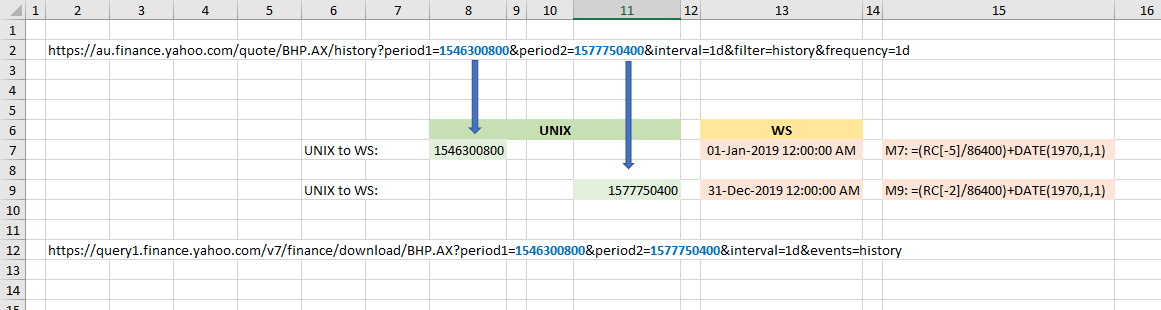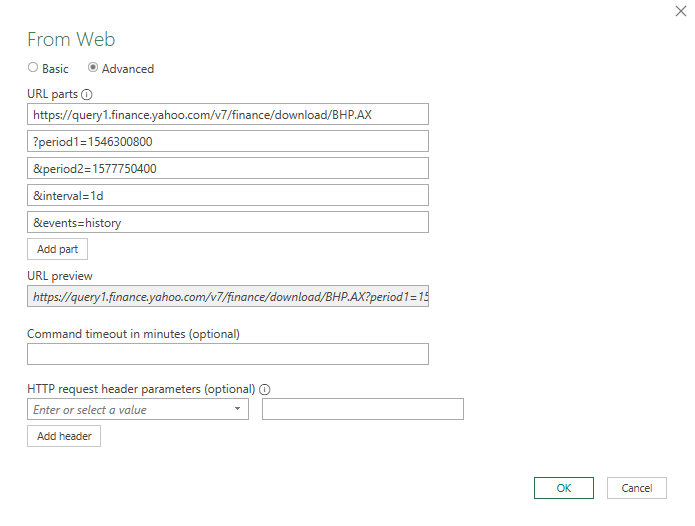# xlf | WS date - UNIX timestamp

UNIX timestamps are commonly used on web based data URL links. Consider the URL at row 2 of the worksheet in figure 1.
https://au.finance.yahoo.com/quote/BHP.AX/history?period1=1546300800&period2=1577750400&interval=1d&filter=history&frequency=1d.

This is a search string applied to Yahoo Finance, for BHP stock traded on the ASX, daily data.

• Period 1 is the start of the data window: UNIX time 1546300800, equivalent to 1 January 2019
• Period 2 is the end of the data window: UNIX time 1577750400, equivalent to 31 December 2019Fig 1: UNIX timestamps - are measured in the number of seconds since 1 January 1970

## Excel date and time

• The Excel epoch is 1 January 1900 with time units of 1 day

## UNIX date and time

• The UNIX timestamp epoch is 1 January 1970 with time units of 1 second
• I January 1970 is Excel serial date 25569, returned by the WS function DATE(1970,1,1)
• An Excel serial day has $24 \times 60 \times 60 = 86400$ seconds

## UNIX ↔ WS conversion

From the example in figure 2, the WS formulas are:

• Convert WS date to UNIX: D5: =(RC[-2] - DATE(1970,1,1))*86400
• Convert UNIX to WS: D18: =(RC[-2]/86400) + DATE(1970,1,1)

## URL string for Excel Web data linkFig 3: URL string - Advanced option for "From Web" item in the Data, Get and Transform data group - historical data (&events=history) at daily intervals (&intervals=1d)